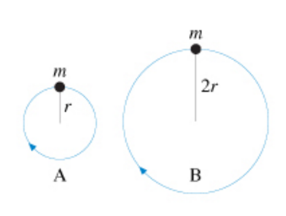# Problem: The figure(figure 1) shows two balls of equal mass moving in vertical circles Is the tension in string A greater than less than, or equal to the tension in string B if the balls travel over the top of the circle with equal speed?a. The tension in string A is greater than the tension in string Bb. The tension in string A is less than the tension in string Bc. The tension in string A is equal to the tension in string B

###### FREE Expert Solution

Centripetal force:

$\overline{){\mathbf{F}}{\mathbf{=}}\frac{\mathbf{m}{\mathbf{v}}^{\mathbf{2}}}{\mathbf{r}}}$

The tension in the string is equal to the centripetal force.

v and m is equal for both.

Centripetal force is inversely proportional to the radius.

79% (351 ratings)###### Problem Details

The figure(figure 1) shows two balls of equal mass moving in vertical circlesIs the tension in string A greater than less than, or equal to the tension in string B if the balls travel over the top of the circle with equal speed?

a. The tension in string A is greater than the tension in string B

b. The tension in string A is less than the tension in string B

c. The tension in string A is equal to the tension in string B